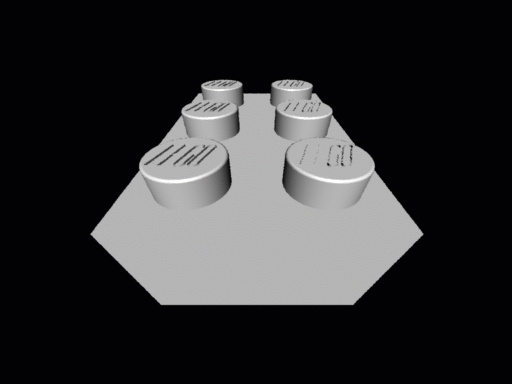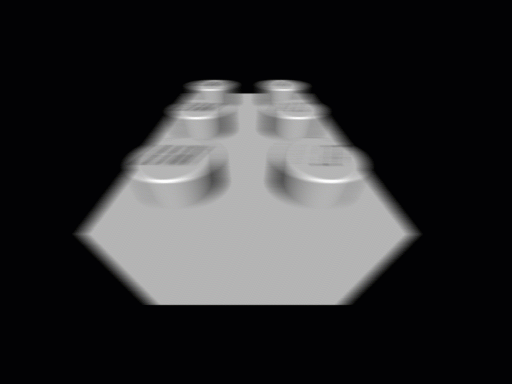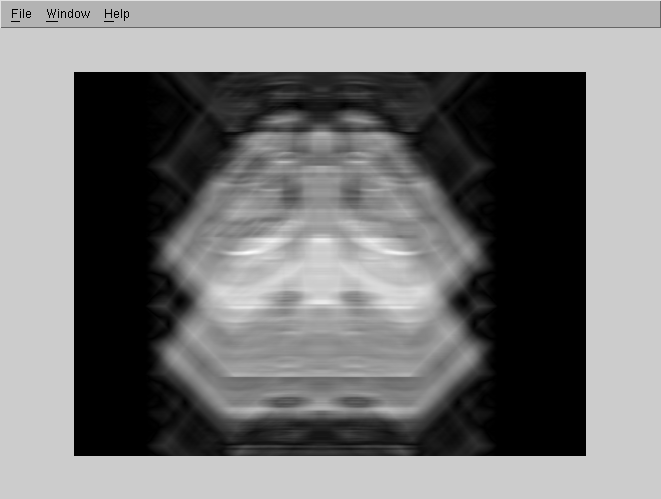Imaging Lab 6
The moving Lego...
Ted Gould and Bryan Evenson

The purpose of this lab was to "un motion blur" an image.  For our original image we used the following picture:Then we used a graphics program called The GIMP to motion blur the image 5 pixels horizontally in linear mode as follows:We then applied the following program to the Fourier transformation of the image in an attempt to get the original image back.

function x = newfunc(im, width, height, moved)

for j = 1:width
for k = 1:height
wval = j - width/2;
if (wval == 0) wval = 1; end;
fpic(j, k) = im(j, k) * (1/(pi * wval * moved) * sin(pi * wval * moved) * exp(- i * pi * wval * moved));
if (fpic(j,k) == 0)
fpic(j,k) = 1;
end;
end;
end;
x = fpic;

And the image that we got with: imshow(mat2gray(abs(ifft2(newfunc(imread('lego.motion.tiff'), 384, 512, 5))))); is:This is the best that we were able to get the image back to it's original.  The image seems to be blurred in the vertical direction instead of it's original bluring in the horizontal direction.  We are curious whether or not this is caused by some artificats of take a continous function and applying it digitally, where the horizontal values may be correct but the vertical ones are approximated.